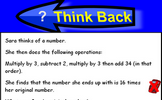# Algebraic representation

### Term 3 starting in week 9 :: Estimated time: 1 weeks

• Draw and interpret quadratic graphs
• Interpret graphs, including reciprocal and piece-wise
• Represent inequalities

For higher-attaining pupils:

• Investigate graphs of simultaneous equations

This page should remember your ticks from one visit to the next for a period of time. It does this by using Local Storage so the information is saved only on the computer you are working on right now.

## Lesson Starters

Here are some suggestions for whole-class, projectable resources which can be used at the beginnings of each lesson in this block.

### 1st Lesson#### Think Back

A problem which can be answered by forming an algebraic equation then solving it.

### 2nd Lesson#### Simultaneous Occasions

A problem which can best be solved as a pair of simultaneous equations.

### 3rd Lesson#### Rail Weigh

Use the weights of the trains to work out the weight of a locomotive and a coach. A real situation which produces simultaneous equations.

Some of the Starters above are to reinforce concepts learnt, others are to introduce new ideas while others are on unrelated topics designed for retrieval practice or and opportunity to develop problem-solving skills.

White Rose ResourcesEnd of block assessments provide a quick progress check at the end of each block of learning to make sure students have understood the content covered. This Scheme of Learning was produced by White Rose Maths and is used here with permission granted on 30th June 2021.For All: